# Transformation of Functions: Dilation (Stretches)

#### Vertical Stretches and Compressions

Given a function $f(x)$, a new function $g(x)=c f(x)$, where $c$ is a positive constant, is a vertical stretch or vertical compression (parallel to the $y$-axis) of the function $f(x)$ with a scale factor $c$.

• If $c>1$, then the graph will be stretched.

• If $0< c< 1$, then the graph will be compressed.

#### Horizontal Stretches and Compressions

Given a function $f(x)$, a new function $g(x)= f(cx)$, where $c$ is a positive constant, is a horizontal stretch or horizontal compression (parallel to the $x$-axis) of the function $f(x)$ with a scale factor $\frac{1}{c}$.

• If $c>1$, then the graph will be compressed by $\dfrac{1}{c}$.

• If $0< c< 1$, then the graph will be stretched $\dfrac{1}{c}$.

#### Question 1

The point $P(3, -2)$ lies on the graph $y=f(x)$. State the coordinates of the map of the point $P$ on each of the following graph .
$\begin{array}{l} \\ \text{(a) } y=f(2x)\\\\ \text{(b) } y=f(2x-1) \\\\ \text{(c) } y= f(2x-1) -3\\\\ \text{(d) } y=3f(x)\\\\ \text{(e) } y=-3f(x)\\\\ \text{(f) } y=-3f(-x)\\\\ \end{array}$

Let the mapped point of $P(3, -2)$ be $P'(a, b)$.
\begin{aligned} &\\ \text{(a) } \quad & \text{ On the curve } y=f(2x),\\\\ &a=\dfrac{1}{2}\times 3 = \dfrac{3}{2}\\\\ &b=-2\\\\ \end{aligned}
The mapped point of $P(3, -2)$ on the curve $y=f(2x)$ is $\left(\dfrac{3}{2},-2\right)$
\begin{aligned} &\\ \text{(b) } \quad & \text{ On the curve } y=f(2x-1),\\\\ &a=\dfrac{1}{2}\times (3 +1) = 2\\\\ &b=-2\\\\ \end{aligned}
The mapped point of $P(3, -2)$ on the curve $y=f(2x)$ is $(2,-2)$
\begin{aligned} &\\ \text{(c) } \quad & \text{ On the curve } y=f(2x-1)-3,\\\\ &a=\dfrac{1}{2}\times (3 +1) = 2\\\\ &b=-2-3=-5\\\\ \end{aligned}
The mapped point of $P(2, -5)$ on the curve $y=f(2x)$ is $(2,-5)$
\begin{aligned} &\\ \text{(d) } \quad & \text{ On the curve } y=3f(x),\\\\ &a=3\\\\ &b=3(-2)=-6\\\\ \end{aligned}
The mapped point of $P(3, -2)$ on the curve $y=f(2x)$ is $(3,-6)$
\begin{aligned} &\\ \text{(e) } \quad & \text{ On the curve } y=-3f(x),\\\\ &a=3\\\\ &b=-3(-2)=6\\\\ \end{aligned}
The mapped point of $P(3, -2)$ on the curve $y=f(2x)$ is $(3,6)$
\begin{aligned} &\\ \text{(d) } \quad & \text{ On the curve } y=-3f(-x),\\\\ &a=-3\\\\ &b=3(-2)=-6\\\\ \end{aligned}
The mapped point of $P(3, -2)$ on the curve $y=f(2x)$ is $(-3,-6)$

#### Question 2

The point $P(-1, 3)$ lies on the graph $y=f(x)$. State the coordinates of the map of the point $P$ on each of the following graph .

 $\text{(a)}$ On the graph of $y=a f(x)$, where $a$ is a positive constant, the point $P$ is mapped to the point $(-1,1)$. Determine the value of $a$. $\text{(b)}$ On the graph of $y={f}(k x)$, where $k$ is a positive constant, the point $P$ is mapped to the point $(-3,3)$. Determine the value of $k$.

 $\text{(a)}$ The point $P(−1,3)$ lies on the graph $y=f(x)$. The mapped point of $P$ on the graph $y=af(x)$ is $(-1, 3a)$. By the problem, the mapped point of $P$ on the graph $y=af(x)$ is $(-1, 1)$. $\therefore\ 3a = 1\Rightarrow a=\dfrac{1}{3}$ $\text{(b)}$ The point $P(−1,3)$ lies on the graph $y=f(x)$. The mapped point of $P$ on the graph $y=f(kx)$ is $\left(-\dfrac{1}{k}, 3\right)$. By the problem, the mapped point of $P$ on the graph $y=af(x)$ is $(-3, 3)$. $\therefore\ -\dfrac{1}{k} = -3\Rightarrow k=\dfrac{1}{3}$

#### Question 3

The point $A(a, b)$ lies on the graph $y=f(x)$. State the coordinates of the map of the point $A$ on each of the following graphs.
$\begin{array}{l} \\ \text{(a) } y=\dfrac{1}{2}f(x) \\\\ \text{(b) } y=3f(x) + 1 \\\\ \text{(c) } y=f(2x-1) \end{array}$

The point $A(a,b)$ lies on the graph $y=f(x)$.
\begin{aligned} &\\ \text{(a) }\ &\text{On the curve } y=\dfrac{1}{2}f(x)\\\\ &\text{The mapped point of } A \text{ is } \left(a,\dfrac{b}{2}\right)\\\\ \text{(b) }\ &\text{On the curve } y=3f(x)+1\\\\ &\text{The mapped point of } A \text{ is } \left(a,3b+1\right)\\\\ \text{(c) }\ &\text{On the curve } y=f(2x-1)\\\\ &\text{The mapped point of } A \text{ is } \left(\dfrac{a+1}{2},b\right)\\\\ \end{aligned}

#### Question 4

The figure shows the parabola with equation y = f ( x ) with vertex at B( 2,4). The graph cuts the x axis at O( 0, 0) and at the point A( 4, 0).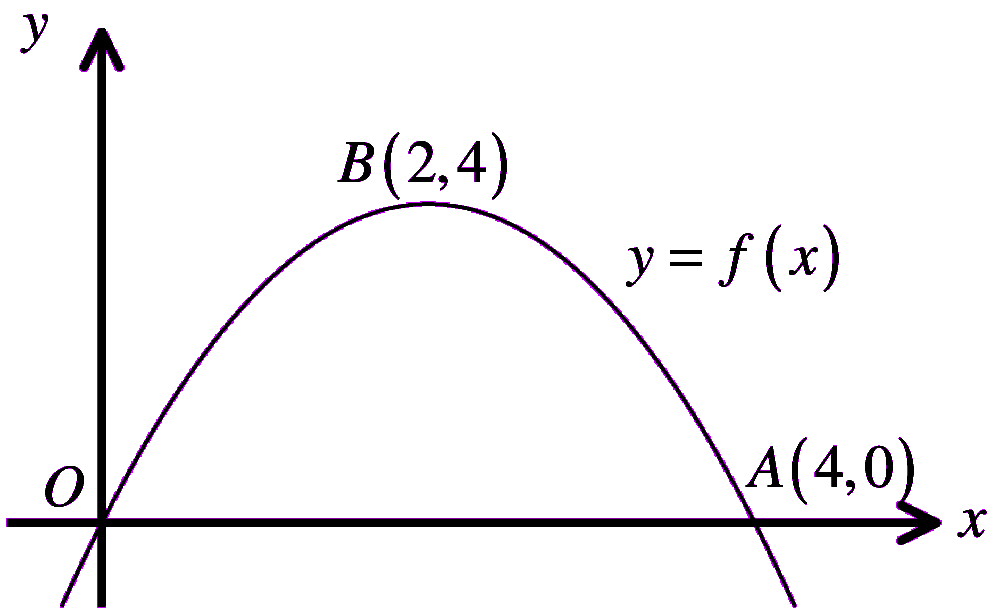Sketch on a separate set of axes the graph of …
\begin{aligned} &\\ &\text{(a) } y=3f(x)\\\\ &\text{(b) } y=f\left(\frac{x}{2}\right)\\\\ \end{aligned}
Each sketch must include the coordinates of any points where the graph crosses the coordinate axes and the new coordinates of the vertex of the curve.

(a)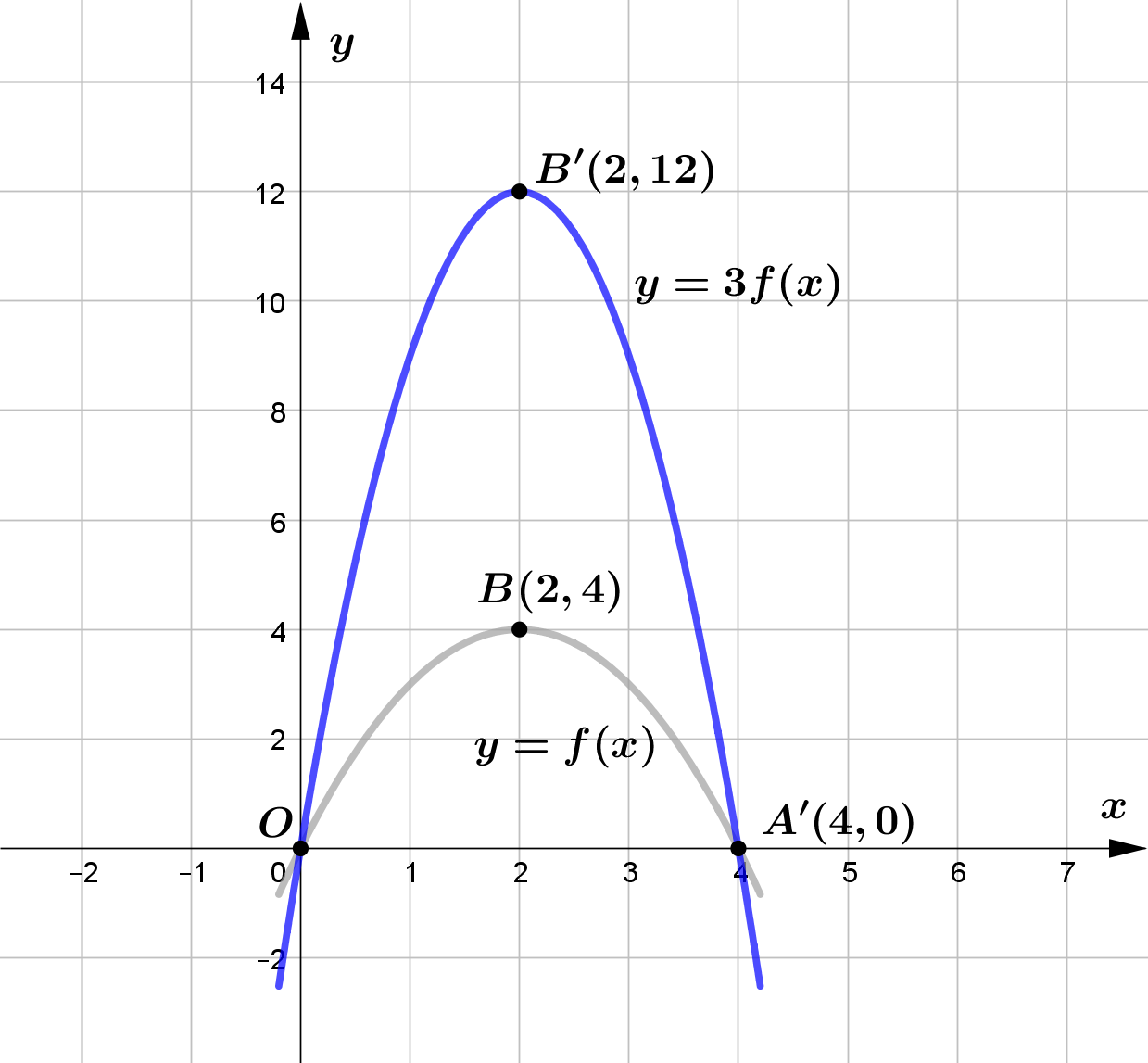(b)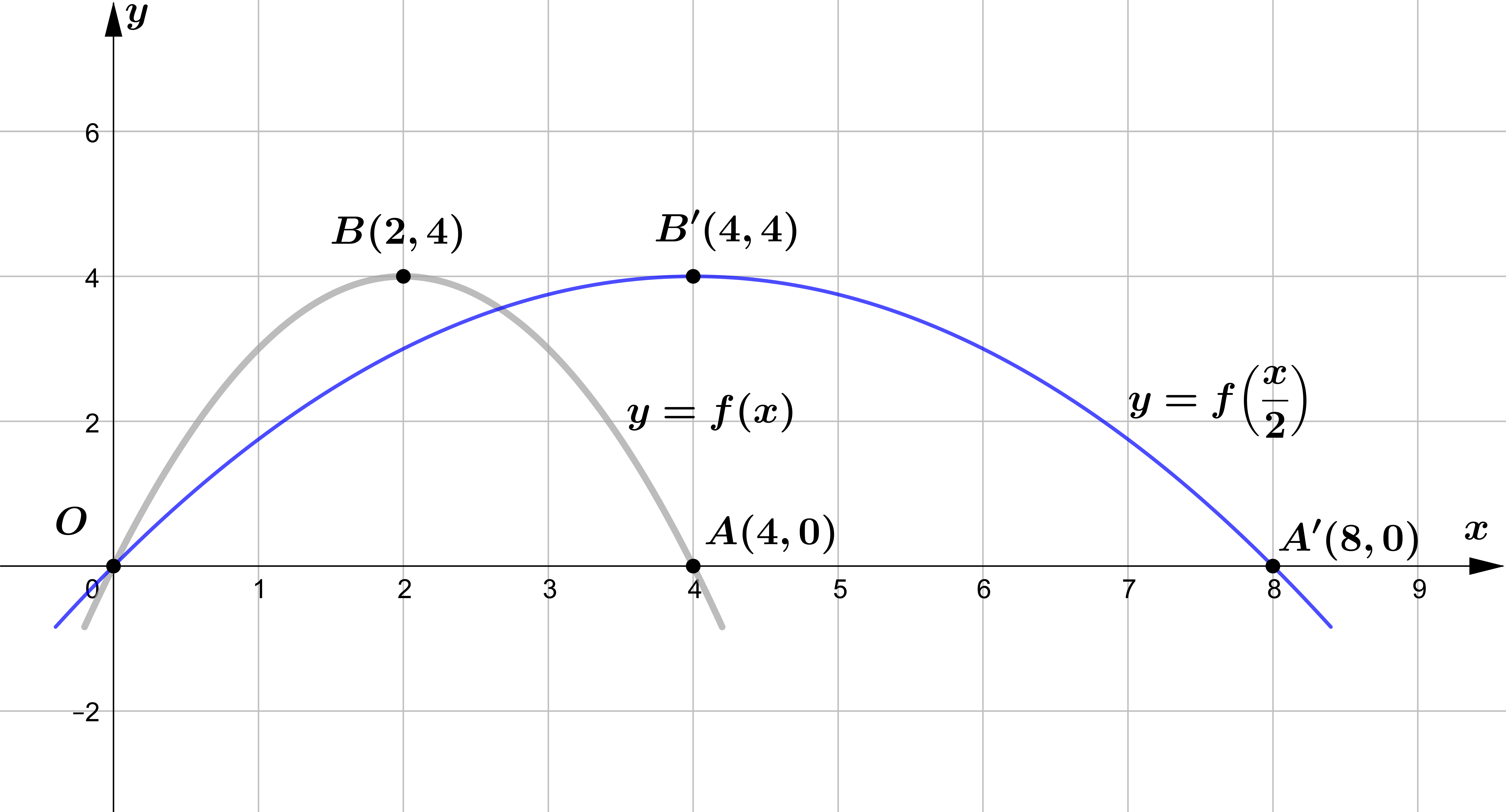#### Question 5

The figure shows a sketch of the curve with equation $y = f(x)$.

The curve passes through the points $(0, 3)$ and $(4, 0)$ and touches the $x$-axis at the point $(1, 0)$.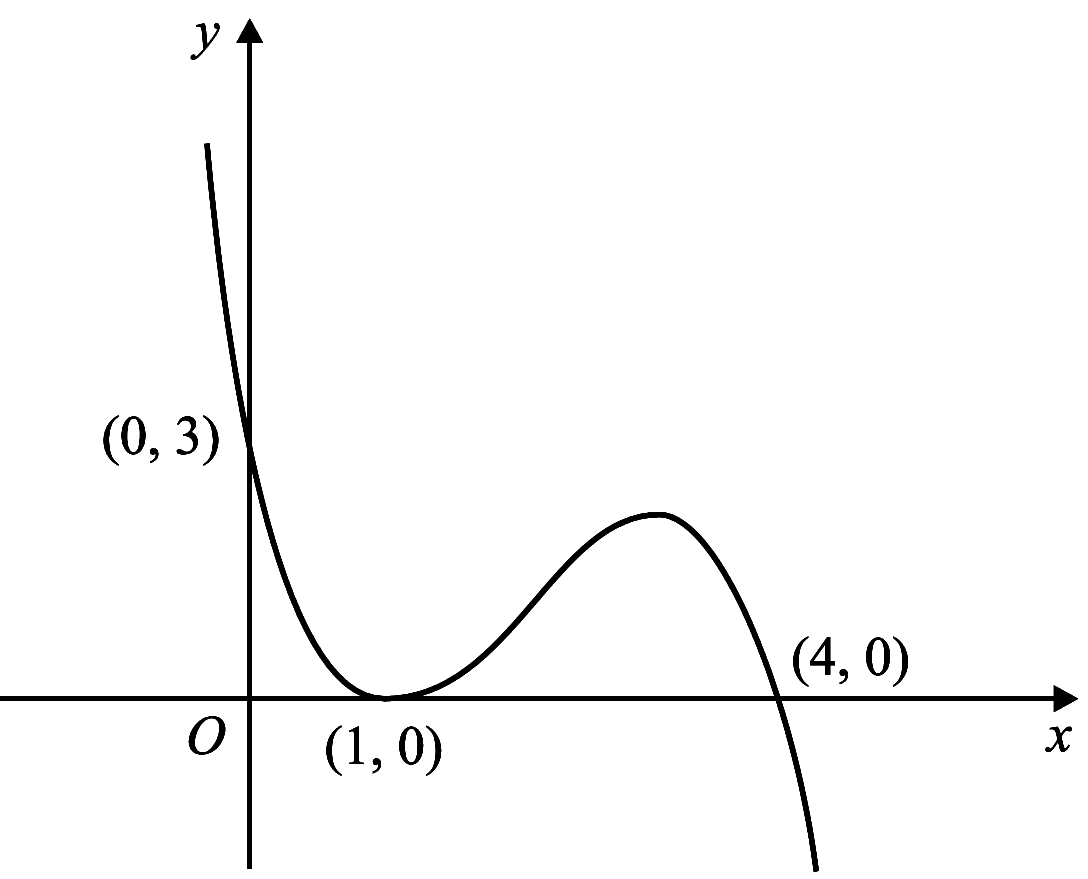On separate diagrams sketch the curve with equation ...
\begin{aligned} &\\ &\text{(a) } y = f(2x)\\\\ &\text{(b) } y = 2f(x)\\\\ &\text{(c) } y = -2f(x)\\\\ \end{aligned}
On each diagram show clearly the coordinates of all the points where the curve meets the axes.

(a)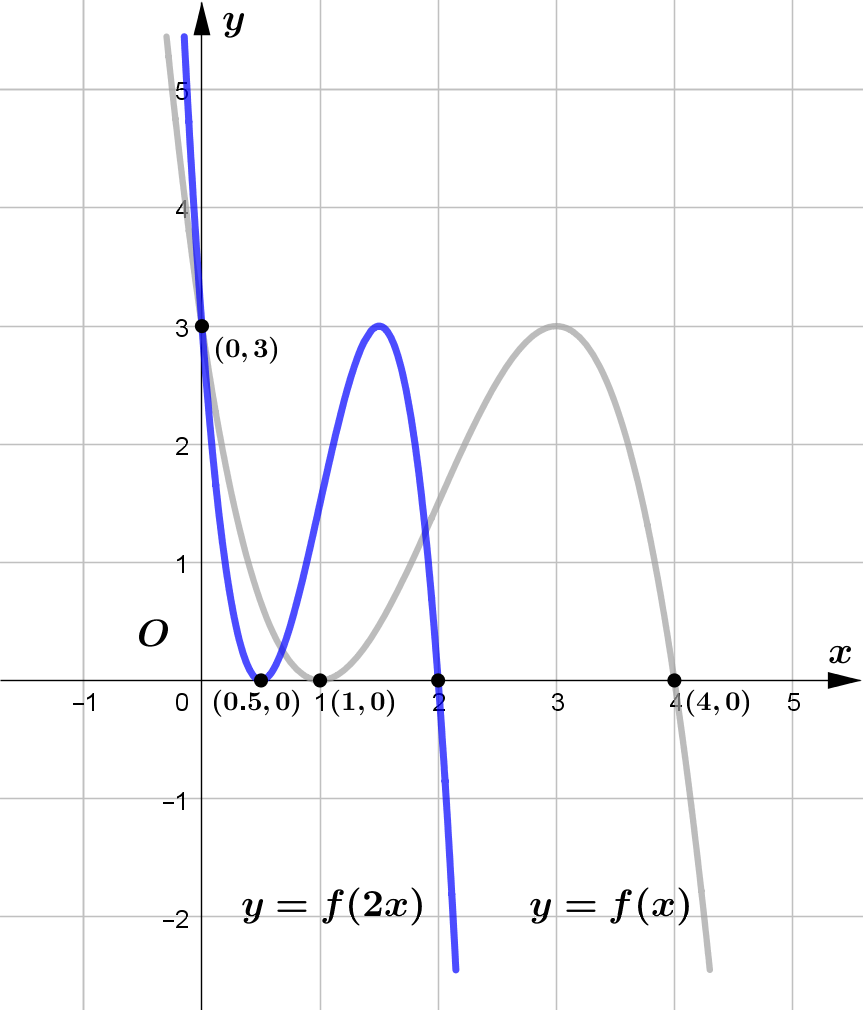(b)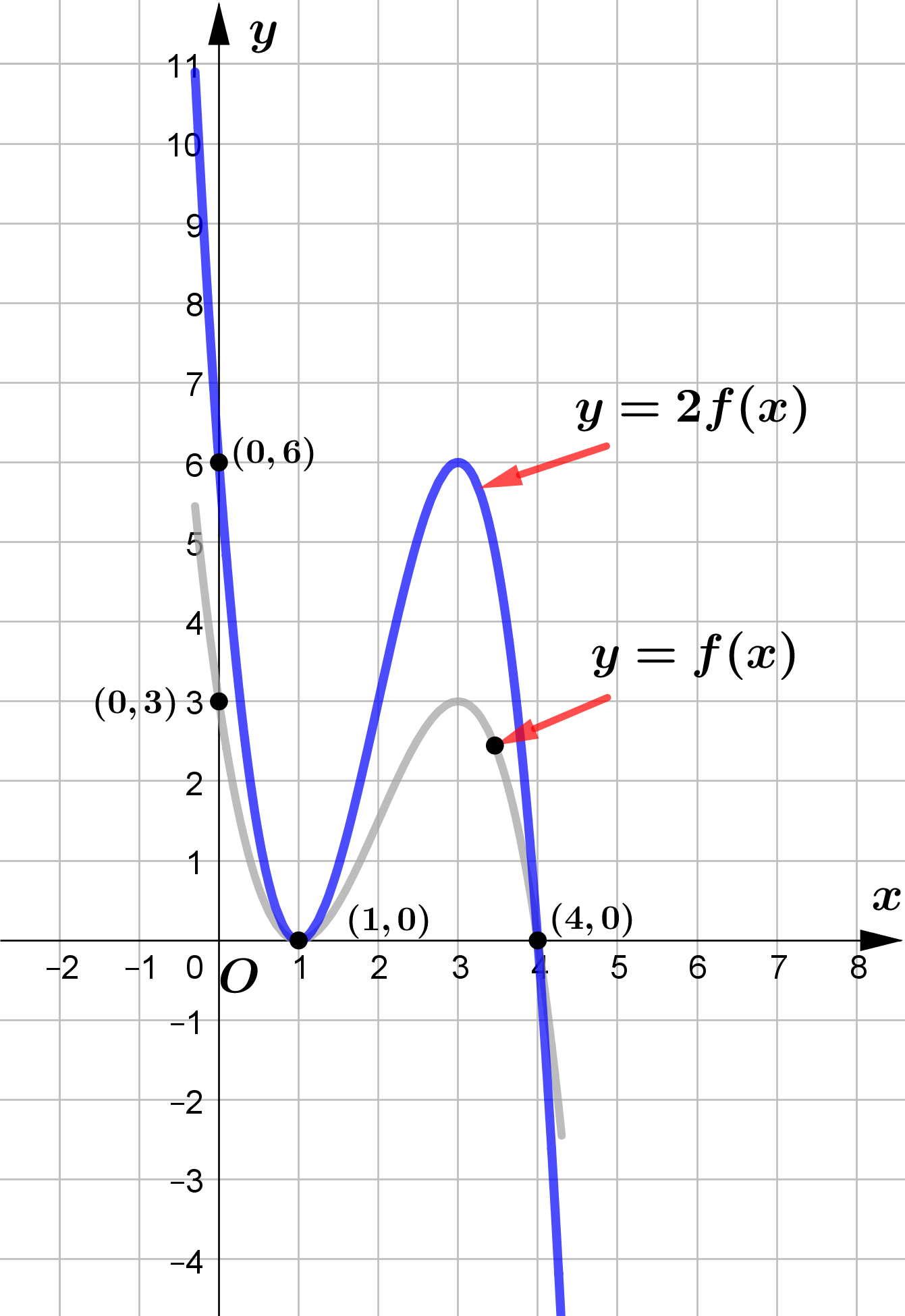(c)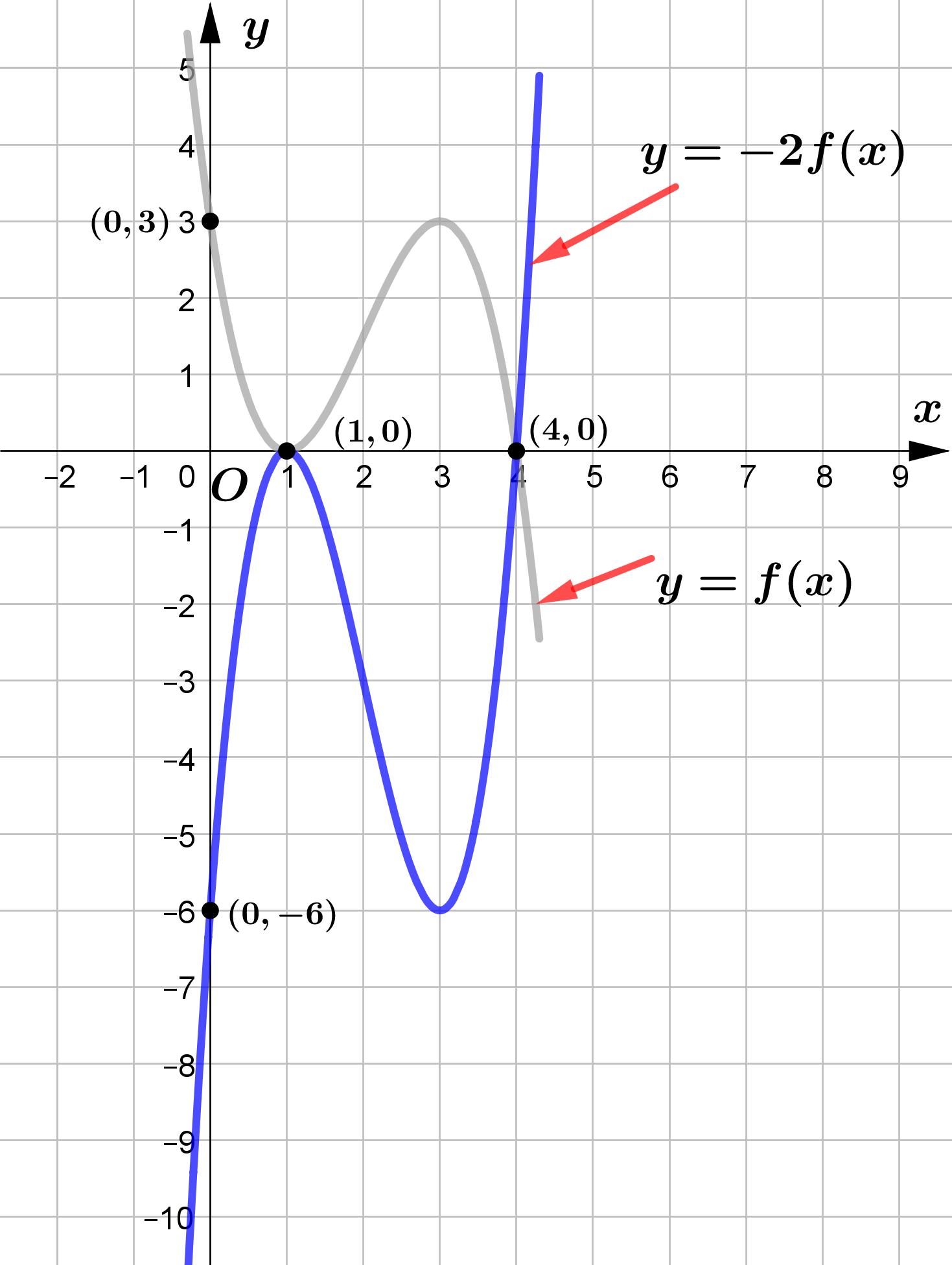#### Question 6

Given that $f(x)=\sqrt{x}$.

The following table represents the coordinate of some points on the graph $y=f(x)$.

$\begin{array}{|c|c|c|c|c|c|} \hline x & 0 & 1 & 4 & 9 \\ \hline y & 0 & 1 & 2 & 3 \\ \hline \end{array}$

 $\text{(a)}$ State the domain and range of $f(x)$. $\text{(b)}$ Use table to sketch the graph of $y=f(x)$. $\text{(c)}$ Describe the transformation that transforms the graph $y=\sqrt{x}$ to the graph $y=\sqrt{x-4}$. $\text{(d)}$ The graph $y=\sqrt{x}$ is stretched by a scale factor of $2$ parallel to the $x$-axis and then it is translated $3$ units right. State the equation of the transformed graph. $\text{(e)}$ The graph $y=\sqrt{x}$ is stretched by a scale factor of $5$ parallel to the $x$-axis. State the equation of the transformed graph. $\text{(f)}$ If $(8,4)$ is a point on the graph $y=f(cx)$, what is the value of $c$?

$\textbf{(a)}$ domain of $f=\{x\mid x\ge 0, x\in\mathbb{R}\}$
$\quad\ \$ range of $f= \{y\mid y\ge 0, y\in\mathbb{R}\}$.

$\textbf{(b)}$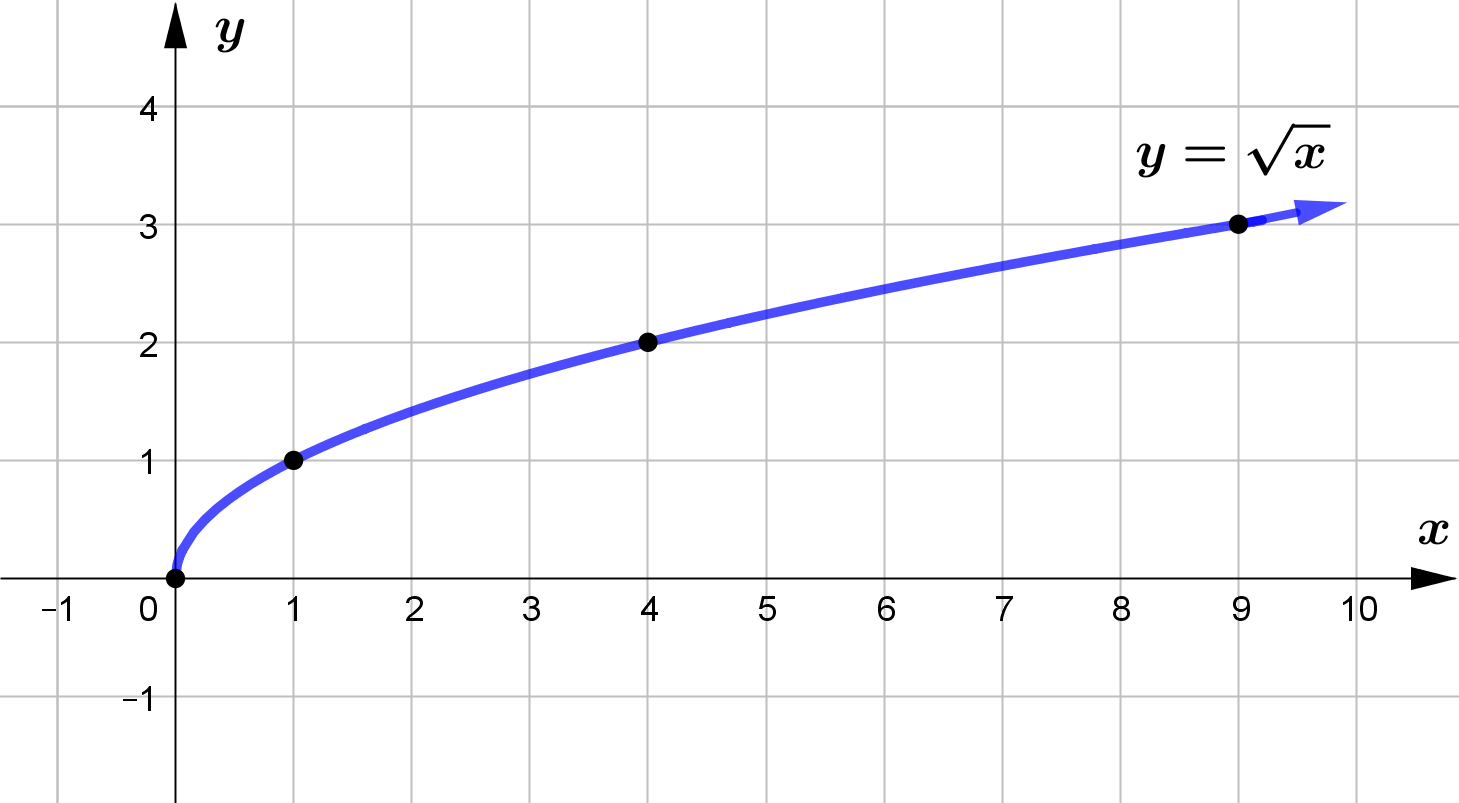$\textbf{(c)}$ The graph of $y=\sqrt{x-4}$ is obtained from $y=\sqrt{x}$ by shifting $4$ units right.

$\textbf{(d)}$ The equation of the transformed graph is $y=\sqrt{\dfrac{1}{2}(x-3)}$

$\textbf{(e)}$ The equation of the transformed graph is $y=\sqrt{\dfrac{1}{5}x}$

$\textbf{(f)}$ The coordinates of any point on the graph $y=f(cx)$ is $(x, \sqrt{cx})$.

By the problem, $(8, 4)$ lies on the graph $y=f(cx)$

\begin{aligned} \therefore\ \sqrt{8c} &= 4\\\\ 8c &= 16\\\\ c&=2 \end{aligned}